﻿ A Stochastic Data Envelopment Analysis Model Considering Variation in Input and Output Variables

### A Stochastic Data Envelopment Analysis Model Considering Variation in Input and Output Variables

Basma E. El-Demerdash, Ihab A. El-Khodary, Assem A. Tharwat

## A Stochastic Data Envelopment Analysis Model Considering Variation in Input and Output Variables

Basma E. El-Demerdash1,, Ihab A. El-Khodary1, Assem A. Tharwat2

1Department of Operations Research and Decision Support, Faculty of Computers and Information, Cairo University, Egypt

2College of Business Administration, American University in the Emirates, United Arab Emirates

### Abstract

Data envelopment analysis (DEA) is a nonparametric method in is used to measure the relative efficiency of comparable institutions and also used for benchmarking in operations management. There is a weakness in conventional DEA models that it does not allow uncertainty variations in input and output variables however, in many real life applications variables are usually vague. As a result, DEA efficiency measurement may be sensitive to such variations. Therefore, in this paper, input oriented model is one of the classic models in DEA going to develop in stochastic DEA that allow some of input and output variables have random in nature. Finally, an illustrative example has been presented.

• Basma E. El-Demerdash, Ihab A. El-Khodary, Assem A. Tharwat. A Stochastic Data Envelopment Analysis Model Considering Variation in Input and Output Variables. International Journal of Data Envelopment Analysis and *Operations Research*. Vol. 2, No. 1, 2016, pp 1-6. http://pubs.sciepub.com/ijdeaor/2/1/1
• El-Demerdash, Basma E., Ihab A. El-Khodary, and Assem A. Tharwat. "A Stochastic Data Envelopment Analysis Model Considering Variation in Input and Output Variables." International Journal of Data Envelopment Analysis and *Operations Research* 2.1 (2016): 1-6.
• El-Demerdash, B. E. , El-Khodary, I. A. , & Tharwat, A. A. (2016). A Stochastic Data Envelopment Analysis Model Considering Variation in Input and Output Variables. International Journal of Data Envelopment Analysis and *Operations Research*, 2(1), 1-6.
• El-Demerdash, Basma E., Ihab A. El-Khodary, and Assem A. Tharwat. "A Stochastic Data Envelopment Analysis Model Considering Variation in Input and Output Variables." International Journal of Data Envelopment Analysis and *Operations Research* 2, no. 1 (2016): 1-6.

 Import into BibTeX Import into EndNote Import into RefMan Import into RefWorks

### 1. Introduction

DEA approach is a system analysis method developed by A. Charnes, et al , the famous American experts on operations research, on the basis of “relative efficiency evaluation” concept and it was called CCR (Charnes, Cooper and Rhodes) model. The CCR model extended by Banker et al.  to obtain a variable returns to scale version of the CCR model called BCC (Banker, Charnes and Cooper) model. This approach mainly applies the linear programming technique, and used the observed effective sample data to make the evaluation of production effectiveness on Decision Making Units (DMUs). The objective of a DEA study is to assess the efficiency of each DMU in relation to its peers. The result of a DEA study is a classification of all DMUs as either “efficient” or “inefficient”. Whereas the basic efficiency definition is the output version refers to the ability of an organization with a set of inputs levels, produce the maximum outputs .

One of the advantages of DEA algorithm is not required explicitly specify a mathematical form for the production function and capable of handling multiple inputs and outputs. However, the most important weakness is the traditional DEA models don’t allow stochastic variation in input and output variables and that influence of some uncertainty factors, such as defects of statistical methods, insufficiencies of information source, and randomness of economic phenomena, which makes the use of traditional DEA models to evaluate the efficiencies become very sensitive (e.g. a DMU which is rated as efficient relative to other DMUs, may turn inefficient if such random variations are considered). Taking in to consideration, recent years have seen a great variety of applications of DEA for use in evaluating the relative efficiencies of many different kinds of entities engaged in many different activities in many different contexts in many different countries . DEA applications have used DMUs of various forms to measure the performance of any comparable entities, such as hospitals, financial services, agricultural, educations, air force, banks, courts, and many more, including the performance of countries, regions, etc.

There are good efforts that have been made recently to handle the randomness in data either stochastic input or stochastic output in DEA model. Cooper et al.  extended DEA model by used chance constrained programming formulations to treating congestion in DEA through dealing with all inputs and outputs have random variation. Subhash Ray  modified the standard DEA model to measure relative efficiency in the presence of random variation in the all outputs produced from given deterministic inputs. Desai et al.  proposed a chance-constrained model of traditional DEA that allows random variations only in all output variables, but input variables are deterministic. Talluri et al.  proposed a stochastic input oriented DEA model for vendor selection that assume inputs are deterministic and outputs are random. Razavyan and Tohidi  proposed a DEA method for ranking stochastic efficient of DMUs, by defining the DMU efficiency via joint randomness comparisons of all inputs and outputs with other comparable DMUs.

Kao and Liu  used stochastic DEA technique to treat the stochastic data and find the distributions of the input/output data of each bank. Khodabakhshi  developed an output oriented super-efficiency model in stochastic DEA that consider all data variables have random nature. Tsionas and Papadakis  developed Bayesian inference techniques for stochastic DEA model that consider All inputs and outputs are random in nature and applied to measure efficiency of the Greek banking system for the period 1993–1999. El-Khodary, I., et al.  developed a stochastic DEA model assume that all input variables are random and all outputs are deterministic.

Azadi and Saen  proposed chance-constrained DEA model that allow stochastic in all data variables to determine the most appropriate third-party reverse logistics to assist the decision makers. El-Demerdash et al.  developed a stochastic input oriented DEA model based on the chance constrained programming to help any organization for evaluating their performance given that some inputs are stochastic and recent of inputs and all outputs are deterministic. Vazhayil and Balasubramanian  focused on the second level of hierarchical multi-objective policy optimization which is optimization utilizing DEA. The model working under assumptions that some of input variables are random and recent inputs and all outputs are deterministic. Azadeh, A., et al.  developed a stochastic DEA model that have all inputs and outputs are random for evaluation and ranking DMUs.

This paper forms one part of a series of continuing research efforts. A previous papers treated the topic of stochastic characterizations of efficiency and inefficiency in DEA using chance constrained method to deal with stochastic variation in constraints to convert them to nonlinear form. See El-Khodary, I., et al.  developed an algorithm to help any comparable organizations for evaluating their performance, the developed algorithm based on the DEA model and working in a stochastic environment under assumptions that all input variables are random and the relation between each DMU for the stochastic input variables are independent. El-Demerdash, B. E., et al.,  developed an algorithm for a stochastic chance constrained input oriented model, where the stochastic inputs are normally distributed, while the remaining inputs and all outputs are deterministic and the relation between the same stochastic input variable through different DMUs is dependent. The paper in hand is an extension to the previous work, where we consider that some of input and output variables have random nature and the remaining input and output variables are deterministic and the relation between the same stochastic input variable and the same stochastic output through different DMUs is dependent.

The rest of the paper is organized as follows. The coming section discusses the methodology of the general DEA model and that for the stochastic DEA model. The third section includes the modified stochastic DEA model, and a hypothetical illustrative example. The paper will end with the customary conclusions and implications for the future.

### 2. Basic Mathematical DEA Model

As mention in the introduction, there are two basic models of DEA, the first called “CCR” model developed by Charnes et al.  working under the concept of constant returns-to-scale (CRS), which means the outputs change in direct proportion to the change in inputs, regardless of the size of the DMU, assuming that the scale of operations does not influence efficiency. The second called “VRS” model developed by Banker et al.  under the concept of variable returns-to-scale, which means changing inputs may not result in proportional changes in outputs, a preferred DEA assumption. Another classification to basic DEA models can be either output orientated or input orientated. In the first case, the DEA method seeks the maximum proportional increase in output production, with input levels held fixed. However, for the input oriented case, the DEA method defines the frontier by seeking the maximum possible proportional reduction in input usage, with output levels held constant, for each DMU.

The basic DEA model is a fractional programming model which measures the relative efficiency of ‘n’ DMUs with ‘J’ inputs and ‘K’ outputs depends on maximizing a production function estimated. This function is a deterministic frontier. For any inputs, the value of the DEA estimate defines the maximum output producible from inputs under all circumstances, . But, in our research, we concern about the VRS input oriented model. Therefore, the relative efficiency score of the pth DMU show below:s.t.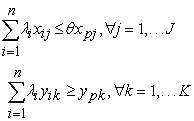(1)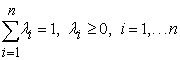Where= efficiency score of DMU p; k = 1 to K (no. of outputs); j = 1 to J (no. of inputs); i = 1 to n ( no. of DMUs);= amount of output k produced by DMU i;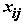= amount of input j utilized by DMU i;= weight given to DMU i.

### 3. Developed a Stochastic DEA Model

Since we interested in evaluating the performance of any comparable institutions to assure the quality given that some of the input and /or output variables might have as stochastic nature, it was necessary to develop a stochastic model deal with this case. Therefore, in this section we present our modification to the stochastic input oriented DEA model (SIODEA) in order to measure relative efficiency in the presence of random variation in some of the outputs . Our developed Stochastic DEA model which is also based on the CCP method is provided below.

3.1. The Chance Constrained input Oriented Data Envelopment Analysis Model

The restriction involving some of input and /or output quantities in the DEA model will be a random inequality that may at times be violated. Because an inequality involving a number of random variables can never be imposed with certainty, the strategy in CCP is to ensure that the probability that the inequality holds for a random sample of these variables does not fall below a certain level. The chance-constrained model for measuring the efficiency level of DMUp is as follow:s.t.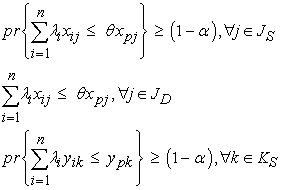(2)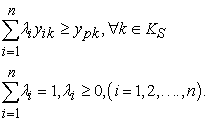where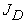set of deterministic inputs,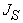set of stochastic inputs,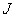set of all inputs, where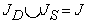and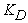set of deterministic outputs,set of stochastic outputs, K set of all outputs, where.

In the SIODEA model developed by El-Demerdash, B. E., et al.  deal with stochastic variation in some of input variables only and the remaining input and all output variables are deterministic. In addition to the authors assumed that each input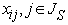is normally distributed with meanand variance; and the relation between the same stochastic input variable through different DMUs is dependent, this means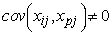. The authors in the SIODEA model using chance constrained method to convert the stochastic linear constraint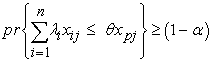(3)

to deterministic nonlinear constraint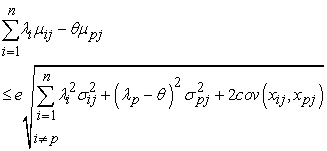(4)

The main goal of our research that develop a stochastic DEA model handle random variations in both input and output variables. So, we add some assumptions on the SIODEA model to handle the stochastic variation in some of outputs and remaining outputs are deterministic variables; in addition to each outputis normally distributed with mean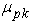and variance; and the relation between the same stochastic output variable through different DMUs is dependent, this means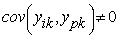.

Accordingly, to pervious assumptions can define the random variable u:(5)

with mean: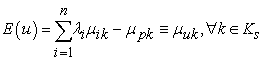(6)

and variance:(7)

Since,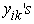are normally distributed, so is variable,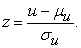(8)

Hence,(9)

Given the symmetry property of normal distribution,(10)

whereis the cumulative standard distribution function. The random inequality restriction in the chance constrained DEA problem can be replaced by the equivalent restriction.(11)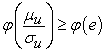(12)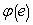is obtained from the table of standard normal distribution. Hence the last equation can be written as,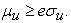(13)

Substitute equations (6) and (7) in equation (13).(14)

Finally, from the above mathematical manipulation, the new presentation for the stochastic DEA model provided in (2) is as shown below: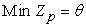s.t.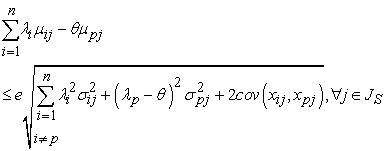(15)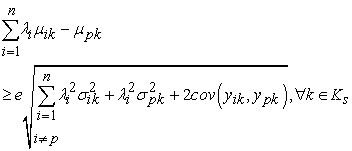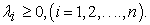### 4. Illustrative Example

The following hypothetical example considers three universities with two input variables which are number of professors in each university (deterministic) and annual budget (stochastic), and three stochastic outputs which are the number of diplomas, bachelors, and masters granted in year by each university. The stochastic variables either input or output are normally distributed with different mean and variance for each university. The data for the deterministic variable is provided in Table 1, while that for the parameters of stochastic variables (mean and variance) are assumed in Table 2, finally Table 3 contains the relation between the same stochastic variable through different university.

#### Table 3. Relation between the same stochastic variable through different university

The aim of this problem is to determine the relative efficiency of each university with respect to each other using the modified model. Assume for the problem that the level of significance for the problem is 5%, and hencewill be 1.96.

As has been explained earlier in model (15), a NLP formulation for each university has to be provided in order to measure the relative efficiency. To evaluate the relative efficiency of university A, we need to solve the following NLP problem:s.t.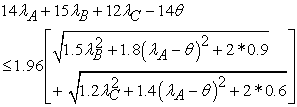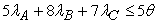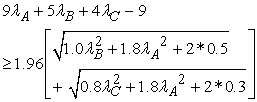(16)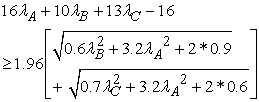The first constraint is responsible for the representation of the stochastic input variable constraint, where the left hand side represents summation of means for each university minus the mean of university A and the right hand side represents the product of the level of significance and square root of summation of the terms variance of each university except university A, variance of university A, and twice covariance between university A and other universities. The second constraint is responsible for the representation of the deterministic input variable constraints, where the left hand side is the summation of input value for each university and the right hand side represents the product of input value of university A and its efficiency level. The third, fourth and fifth constraints are responsible for representing the stochastic output variables constraints, where the left hand side of each constraint represents summation of means for each university minus the mean of university A and the right hand side represents the product of the level of significance and square root of summation of the terms variance of each university except university A, variance of university A, and twice covariance between university A and other universities. Finally, the sixth constraint ensures that the total weights for all DMUs equals to 1. Similarly, the relative efficiency models for university B and C.

We then used the GAMS programming language software to solve the 3 models for each university independently. After running the software the relative efficiency of each university in Table 4 are found. The results reveal that both universities A is efficient, while universities B and C are inefficient.

#### Table 4. Relative Efficiency Scores for each university

For the sake of comparison, our results constructed in the previous paper  used SIODEA model. The relative efficiencies scores for two models SDEA and SIODEA are also provided in Table 5. It could be noticed that the efficient university (university A) remained as is. As for the inefficient universities, university C is turned from inefficient university to efficient university under the SIODEA model, while the remaining university B was still inefficient with a slight improvement in the relative efficiency levels. Therefore, it is obvious that the nature of the variable and hence the constructed model could have an influence on the resulting relative efficiency levels.

### 5. Conclusion and Future Work

DEA is a mathematical optimization method that considered an excellent tool for measuring the performance of comparative DMUs with multiple incommensurate input and output variables. The traditional DEA method requires that the status of all input and output variables be known exactly. However, in many real life applications, the status of some measures is not clearly define as input and/or output variables. These measures are referred to as flexible measures. So, we modified the SIODEA model developed by Demerdash, B. E., et al.  to be deal with some of variables either input or output or both have random nature and the remaining variables are deterministic in nature. Through the illustrative example provided, the developed model showed promising results and comparing its result by the illustrative example provided in the previous work , and the developed model needs to be applied on actual studies.

As part of the future work, it is the intention of the authors to apply the developed model to calculate and compare the relative efficiency of real life application. Further future work, is to develop another input oriented model deal with fuzzy sets in both variables input and output. Finally, the model could then be expanded to include both stochastic and fuzzy uncertainty in variables.

### References

  Charnes A., Cooper W., and Rhodes E. Measuring the efficiency of efficiency of decision-making units. European Journal of Operational Research. 1978, 2(6): 429-444.In article View Article  Banker R., Charnes A., and Cooper W. Some models for estimating technical and scale inefficiencies in data envelopment analysis. Management Science. 1984, 30: 1078-1092.In article View Article  Bandyopadhyay, S. Effect of regulation on efficiency: evidence from Indian cement industry. Central European Journal of Operations Research, 2010, 18(2), 153-170.In article View Article  Cooper, W. W., Seiford, L. M., Zhu, J. Data envelopment analysis: history, models, and interpretations. In Handbook on data envelopment analysis, Springer US. 2011.In article View Article  Cooper, William W., Honghui Deng, Zhimin Huang, and Susan X. Li. Chance constrained programming approaches to congestion in stochastic data envelopment analysis. European Journal of Operational Research. 2004, 155(2): 487-501.In article View Article  Subhash C. Ray. Data Envelopment Analysis: Theory and Techniques for Economics and Operations Research. Cambridge University Press. 2004, 307-325.In article  Desai, A., Ratick, S. J., & Schinnar, A. P. Data envelopment analysis with stochastic variations in data. Socio-Economic Planning Sciences. 2005, 39(2), 147-164.In article View Article  Talluri, S., Narasimhan, R., Nair, A. Vendor performance with supply risk: A chance-constrained DEA approach. International Journal of Production Economics. 2006, 100(2), 212-222.In article View Article  Razavyan, S., Tohidi, G. Ranking of efficient DMUs with stochastic data. In International Mathematical Forum. 2008, 3(2): 79-83.In article  Kao, C., Liu, S. T. Stochastic data envelopment analysis in measuring the efficiency of Taiwan commercial banks. European Journal of Operational Research. 2009, 196(1): 312-322.In article View Article  Khodabakhshi, M. An output oriented super-efficiency measure in stochastic data envelopment analysis: Considering Iranian electricity distribution companies. Computers & Industrial Engineering. 2010, 58(4): 663-671.In article View Article  Tsionas, E. G., Papadakis, E. N. A Bayesian approach to statistical inference in stochastic DEA. Omega. 2010, 38(5): 309-314.In article View Article  El-Khodary, I., El-Demerdash, B., and Tharwat, A. An Algorithm for Evaluating the Performance of Higher Education Organizations in Egypt Using a Stochastic DEA. Proceedings of the 8th International Conference on Data Envelopment Analysis (DEA2010) - Performance Management and Measurement, American University of Beirut, Beirut, Lebanon. 2010, 15-21.In article PubMed  Azadi, M., Saen, R. F. A new chance-constrained data envelopment analysis for selecting third-party reverse logistics providers in the existence of dual-role factors. Expert Systems with applications. 2011, 38(10): 12231-12236.In article View Article  El-Demerdash, B. E., El-Khodary, I. A., and Tharwat, A. A. Developing a Stochastic Input Oriented Data Envelopment Analysis (SIODEA) Model. International Journal of Advanced Computer Science & Applications. 2013, 4(4): 40-44.In article  Vazhayil, J. P., Balasubramanian, R. Optimization of India's power sector strategies using weight-restricted stochastic data envelopment analysis. Energy Policy. 2013, 56: 456-465.In article View Article  Azadeh, A., S. Motevali Haghighi, M. Zarrin, and S. Khaefi. "In article  Performance evaluation of Iranian electricity distribution units by using stochastic data envelopment analysis. International Journal of Electrical Power & Energy Systems 2015, 73: 919-931.In article View Article